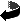# Sobolev spaces on Lie manifolds and regularity for polyhedral domains by Bernd Ammann, Alexandru Ionescu, Victor Nistor

Sobolev spaces on Lie manifolds and regularity for polyhedral domains (.dvi, .ps,.ps.gz oder .pdf)
Doc. Math. 11 161-206 (2006)

### Abstract

We study Sobolev spaces on Lie manifolds, which we define as a class of manifolds described by vector fields. The class of Lie manifolds includes the Euclidean spaces Rn, asymptotically flat manifolds, conformally compact manifolds, and manifolds with cylindrical and polycylindrical ends. As in the classical case of Rn, we define Sobolev spaces using derivatives, powers of the Laplacian, or a suitable class of partitions of unity. We extend the basic results about Sobolev spaces on Euclidean spaces to the setting of Lie manifolds. These results include the definition of the trace map, a characterization of its range, the extension theorem, the density of smooth functions, and interpolation properties. One of the main motivations is that, in the examples we have studied so far, the totally-characteristic Sobolev spaces on polyhedral domains identify with Sobolev spaces on suitable Lie manifolds with boundary.Zurück zur Homepage

The Paper was written on 4.5.2005
Last update 4.7.2006